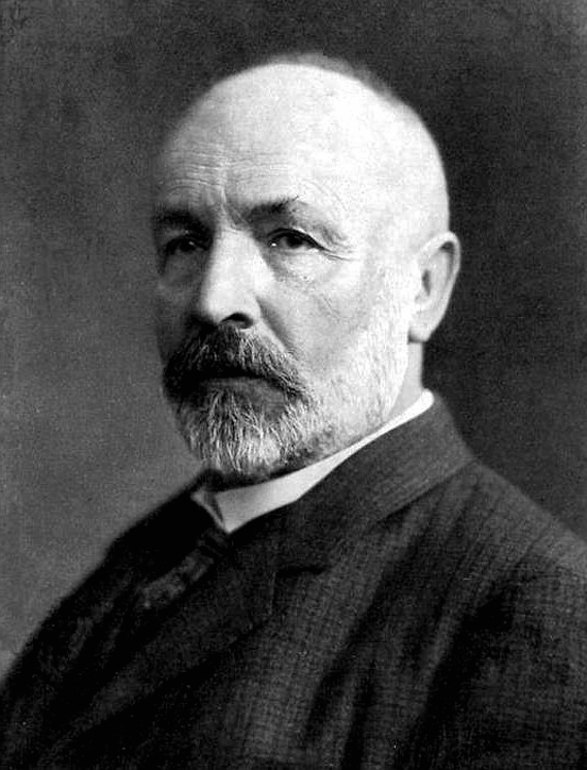# Story

Georg Cantor was the first to make sense of actual, rather than potential, infinity.

Georg Cantor (1845 - 1918), working most of his life in the mathematics department at the University of Halle in eastern Germany, revolutionized the way mathematicians understood infinity.

He was one of the first to treat infinities as actual entities or sizes, and ask questions about the relationship between different infinities.

Before Cantor, infinity was only used by mathematicians as a potential, the limit of a never-ending process.

For example, the Greeks understood that if one considers polygons -- triangle, square, pentagon, hexagon -- inscribed in a circle, and increases the number of sides of the polygon, then "in the limit" as the number of sides "goes to infinity," the area of the polygon "approaches" that of the circle. Ancient mathematicians, including Archimedes, used such limiting processes to calculate many areas and volumes of geometric shapes."In order for there to be a variable quantity in some mathematical study, the domain of its variability must strictly speaking be known beforehand through a definition. However, this domain cannot itself be something variable, since otherwise each fixed support for the study would collapse.

"Thus this domain is a definite, actually infinite set of values. Hence each potential infinite, if it is rigorously applicable mathematically, presupposes an actual infinite."

— Georg Cantor (1886)

After coming to terms with infinities as actual entities, and developing the notion of a one-to-one correspondence between infinite sets, Cantor proved most of the results we discuss on this site.

Since infinity is not a "number" in the usual sense, Cantor coined the term transfinite number to refer to an infinite magnitude. One of his biggest, and most controversial, contributions was the development of transfinite arithmetic -- arithmetic with infinities.

Cantor used the first Hebrew letter, $$\aleph$$, to denote transfinite numbers. So $$\aleph_0$$ is the size of the natural numbers, what we have written as $$|\mathbb{N}|$$. Then $$\aleph_1$$ is the next larger infinity, then $$\aleph_2$$, etc.

Transfinite arithmetic is counterintuitive, and many in the mathematical community objected to Cantor's ideas. For example, in transfinite arithmetic we have the following:

$$\aleph_0 + 1 = \aleph_0$$ (Can you find the one-to-one correspondence?),

$$\aleph_0 \times 2 = \aleph_0$$ (Think of the even and odd integers combining to make the integers.)

$$\aleph_0 \times n = \aleph_0$$ for any integer $$n$$, and

$$\aleph_0 \times \aleph_0 = \aleph_0.$$

The last of these results is equivalent to the statement $$|\mathbb{N}\times\mathbb{N}| = |\mathbb{N}|$$, discussed in this section.

Stating and proving these results rigorously required incredible creativity, imagination, and innovative thinking.

"The essence of mathematics lies entirely in its freedom." -- Georg Cantor

The famous topologist Henri Poincare (1854-1912) later referred to the results of transfinite arithmetic as a "disease" from which mathematics would some day need to be cured, and one of Cantor's old teachers, Leopold Kronecker (1823-1891), called him a "renegade," a "scientific charlatan," and a "corrupter of youth" for teaching infinite set theory. Kronecker said, "I don't know what predominates in Cantor's theory -- philosophy or theology -- but I am sure that there is no mathematics there."

Many arguments -- some mathematical, some philosophical, some theological -- were given for why Cantor must be mistaken. Over time, however, most mathematicians were persuaded by the elegance and clarity of the arguments, and came to welcome Cantor's set theory as fundamental to mathematics. David Hilbert (1862-1943) predicted that "no one would expel us from the paradise that Georg Cantor has opened for us," and the last century has so far shown him correct.

Top